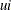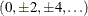# The UNIVARIATE Procedure

### Rounding

When you specify ROUND=u, PROC UNIVARIATE rounds a variable by using the rounding unit to divide the number line into intervals with midpoints of the form, where u is the nonnegative rounding unit and i is an integer. The interval width is u. Any variable value that falls in an interval is rounded to the midpoint of that interval. A variable value that is midway between two midpoints, and is therefore on the boundary of two intervals, rounds to the even midpoint. Even midpoints occur when i is an even integer.

When ROUND=1 and the analysis variable values are between –2.5 and 2.5, the intervals are as follows:

Table 4.27: Intervals for Rounding When ROUND=1

i

Interval

Midpoint

Left endpt rounds to

Right endpt rounds to

–2

[–2.5, –1.5]

–2

–2

–2

–1

[–1.5, –0.5]

–1

–2

0

0

[–0.5, 0.5]

0

0

0

1

[0.5, 1.5]

1

0

2

2

[1.5, 2.5]

2

2

2

When ROUND=0.5 and the analysis variable values are between –1.25 and 1.25, the intervals are as follows:

Table 4.28: Intervals for Rounding When ROUND=0.5

i

Interval

Midpoint

Left endpt rounds to

Right endpt rounds to

–2

[–1.25, –0.75]

–1.0

–1

–1

–1

[–0.75, –0.25]

–0.5

–1

0

0

[–0.25, 0.25]

0.0

0

0

1

[0.25, 0.75]

0.5

0

1

2

[0.75, 1.25]

1.0

1

1

As the rounding unit increases, the interval width also increases. This reduces the number of unique values and decreases the amount of memory that PROC UNIVARIATE needs.